#### Unconstrained mechanical systems

A lattice can even be obtained for the general case of a fully actuated mechanical system, which for example includes most robot arms. Recall from (13.4) that any system in the form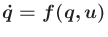can alternatively be expressed as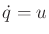, if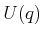is defined as the image of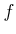for a fixed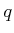. The main purpose of usingis to make it easy to specify a fixed action space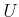that maps differently into the tangent space for each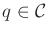.

A similar observation can be made regarding equations of the form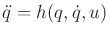, in which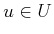andis an open subset of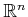. Recall that this form was obtained for general unconstrained mechanical systems in Sections 13.3 and 13.4. For example, (13.148) expresses the dynamics of open-chain robot arms. Such equations can be expressed as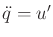by directly specifying the set of allowable accelerations. Eachwill map to a new action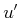in an action space given by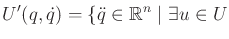such that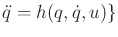(14.24)

for eachand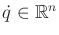.

Each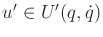directly expresses an acceleration vector in. Therefore, using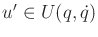, the original equation expressed using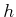can be now written as. In its new form, this appears just like the multiple, independent double integrators. The main differences are

1. The set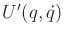may describe a complicated region in, whereasin the case of the true double integrators was a cube centered at the origin.
2. The setvaries with respect toand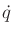. Special concern must be given for this variation over the time sampling interval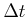. In the case of the true double integrators,was fixed.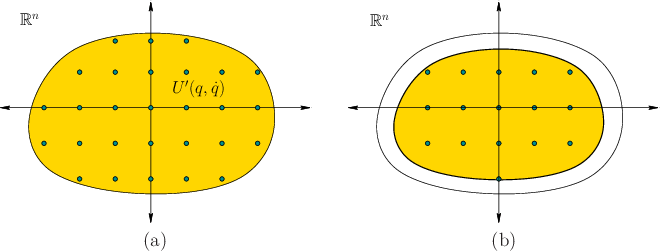The first difference is handled by performing grid sampling overand making an edge in the reachability graph for every grid point that falls into; see Figure 14.15a. The grid resolution can be improved along withto obtain resolution completeness. To address the second problem, think of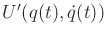as a shape inthat moves over time. Choosingclose to the boundary ofis dangerous because as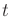increases,may fall outside of the new action set. It is often possible to obtain bounds on how quickly the boundary ofcan vary over time (this can be determined, for example, by differentiatingwith respect toand). Based on the bound, a thin layer near the boundary ofcan be removed from consideration to ensure that all attempted actions remain induring the whole interval. See Figure 14.15b.

These ideas were applied to extend the approximation algorithm framework to the case of open-chain robot arms, for whichis given by (13.148). Suppose thatis an axis-aligned rectangle, which is often the case for manipulators because the bounds for eachcorrespond to torque limits for each motor. Ifandare fixed, then (13.140) applies a linear transformation to obtain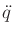from. The rectangle is generally sheared into a parallelepiped (a-dimensional extension of a parallelogram). Recall such transformations from Section 3.5 or linear algebra.

Steven M LaValle 2020-08-14